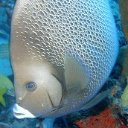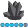WendyT
Pyrite | Level 9

## Is it now possible to send a graph in the body of an E-mail?

Hi folks -

I've been sending plain html reports as attachments to an E-mail for quite awhile, but now would like to send some analysis results.

I was hoping to send a couple SGPLOT graphics and html tables inside an E-mail, with a clickable link to see the whole regression analysis.

Unfortunately, I get the dreaded red X in the E-mail instead of the graphics.

After doing some searching, I came up with usage note 43716 that says that SAS does not support sending graphics under  9.1 TS1M3 SP4.  http://support.sas.com/kb/43/716.html

Has anyone sucessfully done this, or is it still unresolved? (we have 9.2 at the moment)

Wendy T.

5 REPLIES 5

## Re: Is it now possible to send a graph in the body of an E-mail?

Using 9.2, I have sent an email containing an actixeX graph - produced and sent by SAS, needed to change email setting on client to allow viewing...

No special config required on SAs side except the usual SAS email settings,.

Barry

## Re: Is it now possible to send a graph in the body of an E-mail?

can u tell which settting need to change of mailDanH_sas
SAS Super FREQ

## Re: Is it now possible to send a graph in the body of an E-mail?

Hey Wendy,

The best way to send report information over e-mail is by using PDF or RTF. You can attach one file that contains both your graphs and your tables. The example below will generate an example of each using the same style. Check them out and see what you think.

Thanks!

Dan

ods pdf file="test.pdf" style=listing;

ods rtf file="test.rtf" style=listing;

proc sgplot data=sashelp.class;

vbar age / response=height stat=mean;

run;

proc print data=sashelp.class; run;

ods _all_ close;DanH_sas
SAS Super FREQ

## Re: Is it now possible to send a graph in the body of an E-mail?

One other thought:

If it's a requirement that the output be in HTML, you can generate all of your HTML output in one directory and create a ZIP file of that directory for you e-mail. If you use that approach, you should use the PATH option on the ODS HTML statement to set the directory and use the (url=none) to prevent the path from being used by the image references in the HTML page. Your program would look something like the following:

ods html path="c:\temp\MyReport" (url=none) file="TheReport.html";

proc sgplot data=sashelp.class;

vbar age / response=height stat=mean;

run;

proc print data=sashelp.class; run;

ods html close;

Then, just zip up the MyReport directory and e-mail the file.

Thanks!

DanWendyT
Pyrite | Level 9

## Re: Is it now possible to send a graph in the body of an E-mail?

I just tried the pdf and rtf - pdf is probably going to work well if I go with an E-mail attachment.

Now I just have to rethink how I want to do this.My original idea was a nice fitplot in the E-mail itself, with either an attachment or a link to the full regression analysis for those folks that were interested.

The goal is regression (fit) plots with both the nice table of stats and the equation, which is proving quite difficult.

It seems that the regression equation cannot be displayed on the fitplot in PROC REG (and there is one bit of the equation not available when I tried to alter the template).  PROC GPLOT will display the equation, but it's sure not pretty in default.

I have used the OUTEST= option to get the equation parts to put into a PROC SGPLOT reg graphic, but there is no data table like in the fitplot.  I'll do some more reading on ODS to see how I can pull the bits I want from ODS tables.  it just seems strange to have to run REG twice.

At this time, it's two sets of xy variables, but I'm sure that will grow, so am planning on turning this into a macro when I get happy with it.  Any ideas on how to improve this would be very welcome!

Wendy T.

*output directory ;
%LET OUTDIR=&irldata\testing ;
%LET BASE=ss_report ;

*set dependent and independent variables ;
%LET DEPVAR=TSS ;
%LET INDVAR=TURB ;

* set up output ;

ODS LISTING CLOSE ;
FILENAME ODSOUT "&OUTDIR" ;
ods html body="reg-body_&BASE..htm" contents="reg-contents_&BASE..htm" frame="reg-frame_&BASE..htm"  path=ODSOUT gpath=ODSOUT ;
ODS PDF FILE="&outdir.\test.pdf"  ;
ODS RTF FILE="&outdir.\test.rtf"  ;

ODS GRAPHICS ON / RESET=INDEX ;

* do regression with no output to get bits for regression equation ;

PROC REG DATA=START OUTEST=REGSTATS (RENAME= (&INDVAR.=SLOPE))  NOPRINT ;
&DEPVAR._&INDVAR : MODEL &DEPVAR.=&INDVAR ;
RUN ;
QUIT ;

/* Place the regression equation in a macro variable. */
data _null_;
set REGSTATS;
call symput('eqn',"&DEPVAR= "||Intercept||" + "||SLOPE||" * &INDVAR");

*make graphic with inset equation ;
PROC SGPLOT DATA=START ;
REG X=&DEPVAR Y=&INDVAR /CLM CLI  ;

inset "&eqn" / position=bottomright;

footnote1 j=l "Regression Equation";
footnote2 j=l "&eqn";
run;

* run regression again to get the analysis this time with the equation in the footnote ;

PROC REG DATA=START OUTEST=REGSTATS (RENAME= (&INDVAR.=SLOPE _DEPVAR_=DEPVAR)) PLOTS(ONLY LABEL)=(FITPLOT) ;
&DEPVAR._&INDVAR : MODEL &DEPVAR.=&INDVAR ;
RUN ;
QUIT ;

* turn outputs off ;

ODS GRAPHICS OFF ;
ODS _ALL_ close ;
ODS LISTING ;

Discussion stats
• 5 replies
• 6016 views
• 6 likes
• 4 in conversation